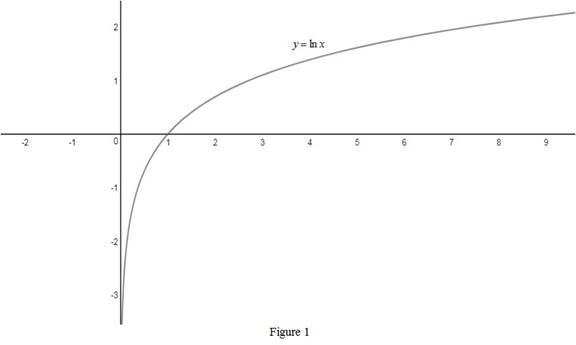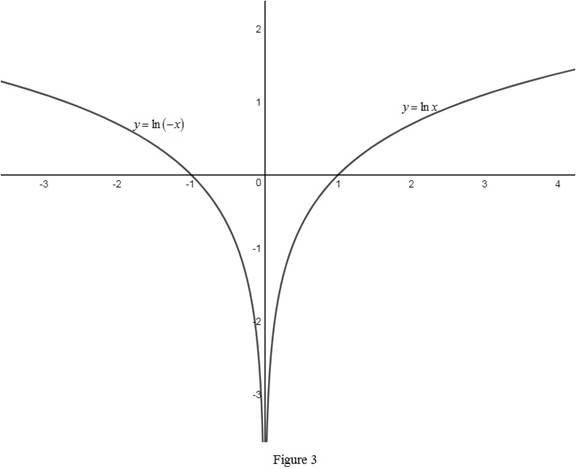# The function y = ln ( − x ) using the graphs from Figure 12 and Figure 13; use the concept of transformations if needed.### Single Variable Calculus: Concepts...

4th Edition
James Stewart
Publisher: Cengage Learning
ISBN: 9781337687805### Single Variable Calculus: Concepts...

4th Edition
James Stewart
Publisher: Cengage Learning
ISBN: 9781337687805

#### Solutions

Chapter 1.6, Problem 48E

(a)

To determine

## To sketch: The function y=ln(−x) using the graphs from Figure 12 and Figure 13; use the concept of transformations if needed.

Expert Solution

### Explanation of Solution

From Figure 13, the graph of the function y=lnx is shown below in Figure 1.Then, draw the graph of y=ln(x) , by reflecting the graph y=lnx about y-axis. Thus, the graph of y=ln(x) is shown below in Figure 2.Observe that Figure 2 is obtained from Figure 1 in such a way that it is reflected about the y-axis.

(b)

To determine

Expert Solution

### Explanation of Solution

The absolute function y=ln|x| is defined as, y={lnxif x>0ln(x)if x<0 .

From part (a), identify that the graph of y=lnx exist when x>0 and the graph y=ln(x) exist when x<0 .

Thus, the graph y=ln|x| is a combination of the graphs y=lnx and y=ln(x) .

Therefore, the graph of y=ln|x| is obtained by drawing the graphs of y=lnx and y=ln(x) on the same screen as shown below in Figure 3.Observe that Figure 3 is obtained from Figure 1 and Figure 2 in such a way that it is drawn on the same screen.

### Have a homework question?

Subscribe to bartleby learn! Ask subject matter experts 30 homework questions each month. Plus, you’ll have access to millions of step-by-step textbook answers!